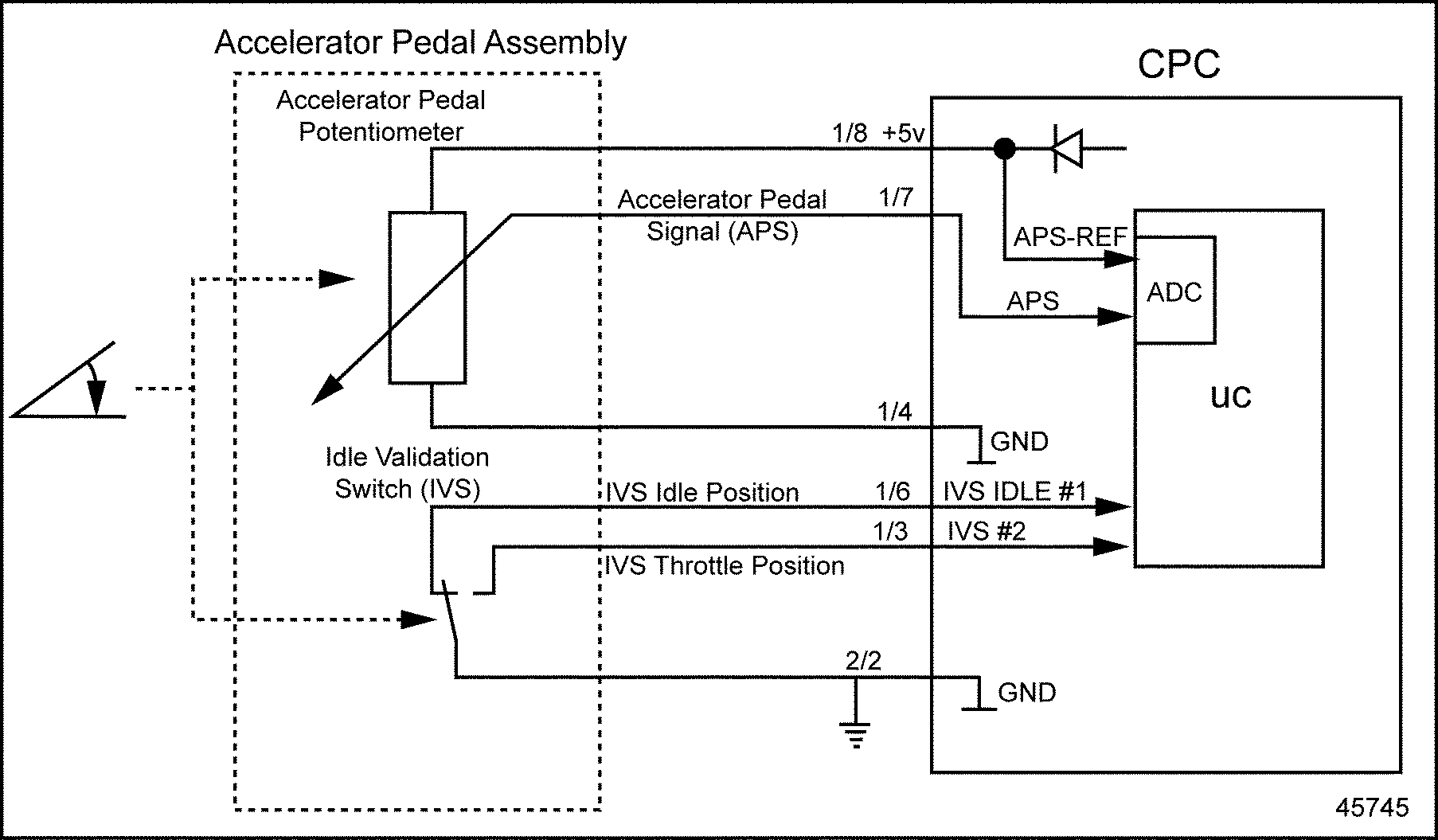# Section 25.3 SPN 91/FMI 4

### Section 25.3 SPN 91/FMI 4

The typical diagnosis for this fault is circuit failed low.‪

#### Section 25.3.1 Low Voltage Check

Perform the following steps to troubleshoot low voltage.‪

1. Disconnect the Accelerator Pedal (AP) connector.
2. Disconnect the CPC #1 connector.
3. Measure the voltage between pins B and C of the AP connector.
1. If the voltage is between 4.5 and 5.5 volts, go to step 5 See Figure "Accelerator Pedal Assembly Installation" .
2. If the voltage is less than 4.5 volts, go to step 4 .
3.Figure 1. Accelerator Pedal Assembly Installation

4. Measure the voltage between pin C of the AP harness connector and ground.
1. If the voltage is between 4.5 and 5.5 volts, repair the open circuit between pin B of the AP harness connector and pin 4 of the CPC #1 connector. Refer to "25.3.1.1 Verify Repairs" .
2. If the voltage is less than 4.5, repair the open circuit between pin C of the AP harness connector and pin 8 of the CPC #1 connector. Refer to "25.3.1.1 Verify Repairs" .
5. Turn OFF the ignition.
6. Disconnect the CPC #1 connector.
7. Measure the resistance between pins B and pin C of the AP connector.
1. If the resistance is less than 5 Ω, repair the short between wires 8 and 4 of the CPC #1 connector. Refer to "25.3.1.1 Verify Repairs" .
2. If the resistance is greater than 5 Ω, go to the next step.
8. Measure the resistance between pin A and B of the AP connector.
1. If the resistance is less than 5 Ω, repair the short between wires 7 and 4 of the CPC #1 connector. Refer to "25.3.1.1 Verify Repairs" .
2. If the resistance is greater than 5 Ω, go to the next step.
9. Measure the resistance between pin C of the AP connector and ground.
1. If the resistance is less than 5 Ω, repair the short circuit between pin C of the AP connector and ground. Refer to "25.3.1.1 Verify Repairs" .
2. If the resistance is greater than 5 Ω, go to the next step.
10. Measure the resistance between pin A of the AP connector and ground.
1. If the resistance is less than 5 Ω, repair the short circuit between pin A of the AP connector and ground. Refer to "25.3.1.1 Verify Repairs" .
2. If the resistance is greater than 5 Ω, replace the Accelerator Pedal. Refer to "25.3.1.1 Verify Repairs" .
##### Section 25.3.1.1 Verify Repairs

Verify repairs as follows:‪

1. Turn ignition OFF.
2. Reconnect any electrical connections that were disconnected to perform the diagnosis.